# Split group

(diff) ← Older revision | Latest revision (diff) | Newer revision → (diff)
over a field,-split group
A linear algebraic group defined overand containing a Borel subgroup that is split over. Here a connected solvable linear algebraic groupis called split overif it is defined overand has a composition series (cf. Composition sequence)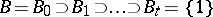such that the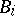are connected algebraic subgroups defined overand each quotient group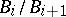is isomorphic overto either a one-dimensional torus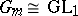or to the additive one-dimensional group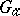. In particular, an algebraic torus is split overif and only if it is defined overand is isomorphic overto the direct product of copies of the group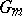. For connected solvable-split groups the Borel fixed-point theorem holds. A reductive linear algebraic group defined overis split overif and only if it has a maximal torus split over, that is, if its-rank coincides with its rank (see Rank of an algebraic group; Reductive group). The image of a-split group under any rational homomorphism defined overis a-split group. Every linear algebraic groupdefined over a fieldis split over an algebraic closure of; ifis also reductive or solvable and connected, then it is split over some finite extension of. Ifis a perfect field, then a connected solvable linear algebraic group defined overis split overif and only if it can be reduced to triangular form over. If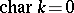, then a linear algebraic group defined overis split overif and only if its Lie algebrais a split (or decomposable) Lie algebra over; by definition, the latter means that the Lie algebrahas a split Cartan subalgebra, that is, a Cartan subalgebra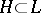for which all eigenvalues of every operator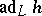,, belong to.
If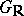is the real Lie group of real points of a semi-simple-split algebraic groupand ifis the complexification of the Lie group, thenis called a normal real form of the complex Lie group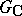.
There exist quasi-split groups (cf. Quasi-split group) over a fieldthat are not split groups over; the groupis an example for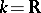.